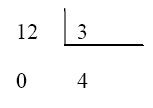# Divisors and multiples of a number

## Divisors of a number

If we take any two integers, for example, $$12$$ and $$3$$, we can do its division:Let us use tables to represent the divisions:

 $$12$$ $$3$$ $$0$$ $$4$$

The resulting quotient, number $$4$$, is an integer. We call this an exact division since the remainder of the division is $$0$$.

Now, if we take any other two numbers, for example, $$12$$ and $$5$$, we can see that they can also be divided:

 $$12$$ $$5$$ $$2$$ $$2$$

In this case, we obtain a remainder, number $$2$$. Therefore, it is not an exact division.

If we try to divide different numbers, we will see that in some cases we obtain exact divisions and in other cases non exact divisions.

If the number $$12$$ is divided by $$1, 2, 3, 4, 6$$ or $$12$$, exact results are obtained, with remainder $$0$$. $$\begin{array}{l} 12\div 1=12 \\ 12 \div 2=6 \\ 12 \div 3=4 \\ 12\div 4= 3 \\ 12\div 6=2 \\ 12 \div 12=1\end{array}$$$It is then said that numbers $$1, 2, 3, 4, 6$$ and $$12$$ are divisors of number $$12$$. So, we would say that one number is a divisor of another one if the division of this second one by the first one (the divisor) results in an exact division. The divisors of number $$10$$ are $$1, 2, 5$$ and $$10$$.$$10 \div 1 = 10 \\ 10 \div 2 = 5 \\ 10 \div 5 = 2 \\10 \div 10 = 1$$$

The divisors of number $$27$$ are $$1, 3, 9$$ and $$27$$.$$21 \div 1=27 \\ 27 \div 3=9 \\ 27 \div 9 = 3 \\ 27 \div 27 =1$$$The divisors of number $$35$$ are $$1, 5, 7$$ and $$35$$.$$35 \div 1=35 \\ 35 \div 5= 7 \\ 35 \div 7=5 \\35 \div 35 =1$$$

The divisors of number $$40$$ are $$1, 2, 4, 5, 8, 10, 20$$ and $$40$$.$$40 \div 1 = 40 \\ 40 \div 2 = 20 \\40 \div 4 =10\\40 \div 5= 8 \\40 \div 8 = 5 \\ 40 \div 10=4 \\ 40 \div 20 =2 \\40 \div 40=1$$$As we see in these examples, the divisors of a number will always be less than this number. Number $$1$$ will always be among them, because any number can be divided by $$1$$. Also, any number will always be a divisor of itself, giving a quotient equal to $$1$$. ## Multiples of a number If we take one of the previous examples, for instance number $$35$$, we can conclude that $$35$$ is a multiple of any of its divisors, which are $$1, 5, 7$$ and $$35$$. A number is a multiple of another one when the latter can be multiplied by a third number to obtain the first one. In this case, we can say, for example, that $$35$$ is a multiple of $$5$$ because: $$5 \times 7=35$$ In the same way, $$35$$ is a multiple of $$7$$, for the same reason. In the other examples, and doing the multiplications of divisors which result is the original number, like $$12, 10, 27$$ or $$40$$, we can conclude that: $$12$$ is multiple of $$1, 2, 3, 4, 6$$ and $$12$$ because all these numbers can be multiplied by another one and give $$12$$ as a result. $$1 \times 12=12 \\ 2 \times 6 = 12 \\ 3 \times 4 =12$$$ $$10$$ is a multiple of $$1, 2, 5$$ and $$10$$. $$1 \times 10 = 10 \\ 2 \times 5 = 10$$$$$27$$ is a multiple of $$1, 3, 9$$ and $$27$$. $$1 \times 27 = 27 \\ 3 \times 9 =27$$$ $$40$$ is a multiple of $$1, 2, 4, 5, 8, 10, 20$$ and $$40$$. $$1 \times 40=40 \\ 2\times 20=40 \\ 40\times 10=40 \\ 5\times 8=40$$\$

As we can see in the examples, any number (other than zero) is a multiple of itself and of the unit (i.e., $$1$$). Also, any number has infinite multiples since it can be always multiplied by any other number